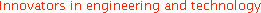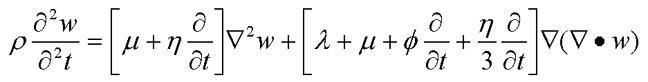Wave3000™ is a stand-alone computer software program for computational ultrasonics for problems that are 3D. It was produced to bring numerical simulation to the ultrasound engineering community. This page provides some basic information about Wave3000™ including a general Program Overview, Hardware/Software/Memory Requirements, and a brief description of the Wave Equation that is being simulated.

Program Overview

Wave3000™ is a stand-alone software package for computational ultrasonics. It operates by solving the three-dimensional (3D) visco-elastic wave equation based on a method of finite differences. The solution is computationally intensive. However, thanks to the ever increasing power and speed of computer hardware, it is now possible to bring such software packages to the desktop and personal computer of the ultrasound engineer and researcher.

Wave3000™ allows the user to compute the full viscoelastic wave solution (both longitudinal and shear displacements) in an arbitrary three-dimensional (3D) object subjected to user-specified acoustic sources. The program, besides simulating the complete spatial and time-dependent acoustic solution, allows the user to simulate ultrasound measurements in a variety of source and receiver configurations. Wave3000™ will prove useful to researchers and applications engineers in such diverse fields as non-destructive testing, materials evaluation, geophysics, medical imaging and biological tissue characterization. The software is useful also in basic mechanistic studies and for academic applications. With Wave3000™ the user can compute solutions (e.g., scattered and reflected waveforms) to virtually an unlimited variety of distinct physical problems without ever leaving the computer keyboard.

Wave3000™ provides solutions to a broad range of 3D ultrasound problems. The program allows the user to specify an arbitrary object which is ultrasonically interrogated. The object is specified in several formats and is based on a voxel representation of the object. Each voxel is associated with a specific material through its value (in the range of 0 to 255). Note that for those users already familiar with Wave2000® (our 2D ultrasound simulation software) or Wave2500™(our 3D-axisymmetric ultrasound simulation software), the similarity of the programs will be apparent in terms of many of the graphical user interfaces.

The 3D object to be simulated can be generated either internally using Wave3000™ "Geometry" routines or externally from other programs (such as 3D images that may be obtained from various scan modalities, for example CT or MRI slice data). Details for this kind of data importing are found in the User Guide and Reference Manual in the Help file.

The attractiveness of Wave3000™ is that it is very easy to generate solutions to a wide variety of 3D ultrasound problems within a simple graphical interface. The user has access to features designed to mimic reasonably closely many practical situations. For example, there are source and receiver configurations that the ultrasound engineer will notice are similar to real ultrasound experimental configurations, for example the use of signals that characterize many standard transducer generated waveforms. As one application, Wave3000™ may be useful for analyzing ultrasound propagation in fluid saturated porous solids, to explore the relationship between porosity and velocity, for example.

Wave3000™ has the potential to generate new insights and approaches to many problems in ultrasonics. For the first time, the user has the capability to "experiment" to his or her heart's content, without turning on a pulser-receiver or connecting a "BNC" cable. Indeed, the simulations can "run" while the user is word processing a document or analyzing data from an earlier simulation. The computer can work "around the clock" (for example, by using the "Multi-batch feature") computing solutions to problems that are difficult to perform in the laboratory or the field, but the results of the simulations can provide important understanding and knowledge for future experiments or for data already collected.

##### Back to the top

Hardware, Software and Memory Requirements

Wave3000 is designed to run on any PC that has the Windows XP, Vista, 7 or 8 operating systems installed. Minimum hardware requirements are extremely modest (for example 15 megabytes free hard disk space and 128 megabytes memory), although we recommend 1 GB or more memory for handling the larger simulation models. (Detailed information on memory requirements can be found in the 'Algorithm' topic of the Wave3000® User Guide Section of the Help file.) Minimum graphics requirements are 256 color VGA and a compatible mouse, but of course most systems will have hardware characteristics much higher than these minimums. As noted, Wave3000® operates best with as much RAM memory as possible, which allows problems of increasingly larger size to be accommodated and avoids the need for virtual (disk) memory to be used. Note also that Wave3000® supports multiprocessor systems, that allows the program to potentially speed-up the execution of the program. This is most effective for "large" objects; experimentation by the user will determine when multiprocessors can lead to faster execution times. The multiprocessors can be either actual multiproccessor systems, or multicore processors, or both.

Wave3000™ is a "memory-hungry" program; this is simply the nature of the wave propagation problem which is being simulated. To aid the user in assessing the memory requirements for a particular simulation model, we can provide the following approximate relationships.

Because of the general nature of the 3D object, the memory requirements must be computed based on the 3D dimensions. The simplest approach for approximating memory needed is to multiply the number of finite difference grid voxels, say N, by 42; this is the amount of memory required in bytes (plus some additional "fixed" program overhead which can usually be neglected in comparison to the 42 x N quantity). As an example, if an object is 2 cm x 3 cm x 4 cm and the voxel resolution ("Voxels/mm") is 10 voxels/mm, the number of voxels in this image will be 200 x 300 x 400 = 24,000,000 voxels. Now if we assume that the finite difference grid elements generated by Wave3000™ are coincident with the number of image voxels (i.e., Grid/Voxle = 1), then the memory required is 42 x N = 42 x 24,000,000 = 960 megabytes.

Another perspective on memory requirements can be gained by determining the memory needed as a function of object size in terms of wavelengths. In the example above, it was assumed that the grid size was coincident with object voxel size, i.e., both were 0.1 mm (10 voxels/mm). For the case of a problem in which the minimum wavelength is about 1 mm, this 0.1 mm size for the finite difference grid element should provide a reasonably accurate solution. We may extend this reasoning for a more generic assessment of memory requirements as follows. Assuming that we would like to have a grid voxel element dimension 10 times less than the minimum wavelength, then that implies that 1,000 x 42 = 42,000 bytes for a cubic object 1 wavelength on a side. If one has a cubic object which is 3 wavelengths on a side, then using the same relative grid dimensions, Wave3000 would require about 1.1 MB of memory. One may also extend this approximation to any number of (minimum) wavelengths to evaluate memory requirements. Assuming a cuboid object Q wavelengths by R wavelengths by S wavelengths in overall dimensions, and again assuming 10 grid elements per wavelength, then the memory required for this model is approximately 42 x 10,000 x Q x R x S = 42 x Q x R x S kilobytes. It is useful to also point out that the minimum wavelength is inversely proportional to the frequency of the source waveform. Therefore, if a simulation with a 1 MHz source waveform requires 1 megabyte of memory, then changing to a source waveform operating at 2 MHz will generally require eight times as much memory, or in this case 8 megabytes, to be used. (This assumes of course that the same size object is used in both cases.) Thus the user may want to carry out as many of his or her simulations as possible with relatively low frequency sources, in order to reduce computational overhead. It is important to point out that there may be instances where the original object geometry has structural features (voxel sizes) smaller than a grid size based on wavelength considerations alone; in this case the user may need to manually reduce the grid size in order to maintain the structural details present in the original image. If this is done, the memory requirements will be larger than the calculations made in the above paragraph, and the user should use the first expression, namely 42 x N, to evaluate the memory needed.

##### Back to the top

Wave Equation

The specific visco-elastic wave equation that is simulated in a Wave3000™ simulation is given by:In the above equation, which applies in an isotropic elastic region,

ρ = material density [kg/m^3],

λ = first Lame constant [N/m^2],

μ = second Lame constant [N/m^2],

η = shear viscosity [N-s/m^2],

φ = bulk viscosity [N-s/m^2],

∇ • = the divergence operator,

denotes the partial differential operator,

t = time [s],

and

w(x,y,z,t) is a three-dimensional column vector whose components are the x, y and z components of displacement of the medium at location (x,y,z), that is

w = [wx(x,y,z,t) wy(x,y,z,t) wz(x,y,z,t]'

where ' denotes matrix transpose. Wave3000 solves the above equation within each homogenous cubic grid element of the object, and computes the displacement (longitudinal and shear) vectors at the intersection of each and every cubic grid element at each time step of the simulation. As noted above, the simulation explicitly satisfies the stress and displacement boundary conditions across each grid element of the simulation model. Additional information on the acoustic wave equation including the viscous loss component (i.e., the viscosity tensor) can be found in the excellent book by B. A. Auld entitled Acoustic Fields and Waves in Solids, Vols. 1-2, 2nd Edition, Krieger Publishing Company, Malabar, Florida, 1990.

Wave3000™ does not implement "ray-tracing" or other "non-general" methods in simulating ultrasound measurements. Rather, it is a comprehensive engineering software package designed to compute the full and accurate solution to practically any 3D ultrasonic problem. Wave3000™ simulates data that you would measure on the lab bench or in the field. In addition, it has an easy to use graphical user interface allowing you to begin simulating complex ultrasound problems in a matter of minutes after installing your Wave3000™ software.

Back to the top